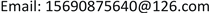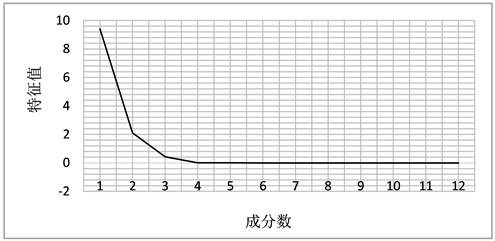﻿ 基于一种新的特征选择方法的朴素贝叶斯分类器选择证券的研究 Research on Security Selection by Naive Bayes Classifier Based on a New Feature Selection Method

Advances in Applied Mathematics
Vol. 08  No. 01 ( 2019 ), Article ID: 28390 , 9 pages
10.12677/AAM.2019.81005

Research on Security Selection by Naive Bayes Classifier Based on a New Feature Selection Method

Panpan Guo, Haijun Liu, Shuangshuang Li

School of Mathematics and Statistics, Zhengzhou University, Zhengzhou HenanReceived: Dec. 18th, 2018; accepted: Jan. 2nd, 2019; published: Jan. 9th, 2019ABSTRACT

In this paper, a naive Bayes classifier for securities selection based on a new feature selection method is established. Firstly, in consideration of the trading data of 50 companies in Shenzhen Stock Exchange and 18 commonly used indicators, a new feature selection method, i.e. the combination of mutual information and principal component analysis, is adopted to select the value factors for classification. Secondly, a naive Bayes classifier is constructed with the data of the first 10 months, and the prediction accuracy of the classifier is tested with that of the last two months. The empirical analysis shows that the average accuracy of the classifier reaches 75%, which is of application value.

Keywords:Feature Selection, Mutual Information, Principal Component Analysis, Naive Bayes ClassifierCopyright © 2019 by author(s) and Hans Publishers Inc.1. 引言

2. 预备知识

2.1. 特征选择

2.2. 互信息

$H\left(X\right)=-\underset{x\in X}{\sum }p\left(x\right)\mathrm{log}p\left(x\right)$ (1)

$H\left(X|Y\right)=-\underset{y\in Y}{\sum }\underset{x\in X}{\sum }p\left(x,y\right)\mathrm{log}p\left(x|y\right)$ (2)

$I\left(X;Y\right)=H\left(X\right)-H\left(X|Y\right)$ (3)

2.3. 主成分分析

2.3.1. 基本概念

1) ${{a}^{\prime }}_{i}{a}_{i}=1$ $\left(i=1,2,\cdots ,p\right)$

2) 当 $i>1$ 时， ${{a}^{\prime }}_{i}\Sigma {a}_{j}=0$ $\left(j=1,2,\cdots ,i-1\right)$

3) $Var\left({Y}_{i}\right)=\underset{{a}^{\prime }a=1,{{a}^{\prime }}_{i}\Sigma {a}_{j}=0\left(j=1,\cdots ,i-1\right)}{\mathrm{max}}Var\left({a}^{\prime }X\right)$

${Y}_{i}={{a}^{\prime }}_{i}X={a}_{1i}{X}_{1}+{a}_{2i}{X}_{2}+\cdots +{a}_{pi}{X}_{p}$ $\left(i=1,2,\cdots ,p\right)$ (4)

2.3.2. 具体步骤

1) 用Z-score法对数据进行标准化变换

2) 求指标数据的相关矩阵

3) 求相关矩阵的特征根与特征向量

4) 计算主成分贡献率及累计贡献率，确定主成分(一般取累计贡献率为85%~95%的特征值所对应的主成分。)

2.4. 朴素贝叶斯分类器

2.4.1. 基本概念

2.4.2. 朴素贝叶斯分类器

${v}_{MAP}=\underset{{v}_{j}\in \left\{{v}_{1},{v}_{2},\cdots ,{v}_{m}\right\}}{\mathrm{arg}\mathrm{max}}\frac{P\left({x}_{1},{x}_{2},\cdots ,{x}_{n}|{v}_{j}\right)P\left({v}_{j}\right)}{P\left({x}_{1},{x}_{2},\cdots ,{x}_{n}\right)}$ (5)

$=\underset{{v}_{j}\in \left\{{v}_{1},{v}_{2},\cdots ,{v}_{m}\right\}}{\mathrm{arg}\mathrm{max}}P\left({x}_{1},{x}_{2},\cdots ,{x}_{n}|{v}_{j}\right)P\left({v}_{j}\right)$ (6)

${v}_{NB}=\underset{{v}_{j}\in \left\{{v}_{1},{v}_{2},\cdots ,{v}_{m}\right\}}{\mathrm{arg}\mathrm{max}}P\left({v}_{j}\right)\underset{i}{\prod }P\left({x}_{i}|{v}_{j}\right)$ (7)

3. 数据，指标与因子

3.1. 数据

3.2. 指标

3.2.1. 股票收益率

${R}_{i,T}=\mathrm{ln}\left(\frac{{P}_{i,T+\Delta t}+{I}_{i,T+\Delta t}}{{P}_{i,T}}\right)$ (8)

3.2.2. 日换手率

3.3. 因子的选取Table 1. Mutual information outcomes of the top five companiesFigure 1. Eigevalues

$\begin{array}{l}{Y}_{1}=0.298643431{Z}_{1}+\cdots +0.273257891{Z}_{11}+0.261699545{Z}_{18}\\ {Y}_{2}=0.206278919{Z}_{1}-\cdots -0.336067968{Z}_{11}-0.494679098{Z}_{18}\end{array}$

4. 构建朴素贝叶斯分类器

5. 结论

Research on Security Selection by Naive Bayes Classifier Based on a New Feature Selection Method[J]. 应用数学进展, 2019, 08(01): 41-49. https://doi.org/10.12677/AAM.2019.81005

1. 1. Fama, E.F. and French, K.R. (1992) The Cross-Section of Expected Stock Returns. The Journal of Finance, 47, 427-465. https://doi.org/10.1111/j.1540-6261.1992.tb04398.x

2. 2. 唐文慧. 基于数据挖掘技术的股价预测实证分析[D]: [硕士学位论文]. 成都: 西南财经大学, 2009.

3. 3. 雷炜, 叶东毅. 利用决策树技术对股票价格数据库进行数据挖掘[J]. 福建电脑, 2004(8): 52-53.

4. 4. 王领, 胡扬. 基于C4.5决策树的股票数据挖掘[J]. 计算机与现代化, 2015(10): 21-24.

5. 5. 钱颖能, 胡运发. 用朴素贝叶斯分类法选股[J]. 计算机应用与软件, 2007, 24(6): 90-92.

6. 6. 左辉, 楼新远. 基于贝叶斯分类的选股方法[J]. 电脑知识与技术, 2008, 2(10): 173-176.

7. 7. 骆桦, 张喜梅. 基于贝叶斯分类法的股票选择模型的研究[J]. 浙江理工大学学报(自然科学版), 2015, 33(3): 418-422.

8. 8. White, H. (1988) Economic Prediction Using Neural Networks: The Case of IBM Daily Stock Returns. IEEE International Conference on Neural Networks, 2, 451-458.

9. 9. Oliveira, F.A.D., Nobre, C.N. and Zárate, L.E. (2013) Applying Artificial Neural Networks to Prediction of Stock Price and Improvement of the Directional Prediction Index—Case Study of PETR4, Petrobras, Brazil. Expert Systems with Applications, 40, 7596-7606. https://doi.org/10.1016/j.eswa.2013.06.071

10. 10. Zahedi, J. and Rounaghi, M.M. (2015) Application of Artificial Neural Network Models and Principal Component Analysis Method in Predicting Stock Prices on Tehran Stock Ex-change. Physica A Statistical Mechanics & Its Applications, 438, 178-187. https://doi.org/10.1016/j.physa.2015.06.033

11. 11. Qiu, M., Song, Y. and Akagi, F. (2016) Application of Artificial Neural Network for the Prediction of Stock Market Returns: The Case of the Japanese Stock Market. Chaos, Solitons & Fractals, 85, 1-7. https://doi.org/10.1016/j.chaos.2016.01.004

12. 12. Almuallim, H. and Dietterich, T.G. (1991) Learning With Many Irrelevant Features. Proceedings of the 9th National Conference on Artificial Intelligence, Anaheim, 14-19 July 1991, AAAI Press, Volume 2.

13. 13. Domingos, P. and Pazzani, M. (1997) On the Optimality of the Simple Bayesian Classi-fier under Zero-One Loss. Machine Learning, 29, 103-130. https://doi.org/10.1023/A:1007413511361

14. 14. Blum, A.L. and Langley, P. (1997) Selection of Relevant Features and Examples in Machine Learning. Artificial Intelligence, 97, 245-271. https://doi.org/10.1016/S0004-3702(97)00063-5

15. 15. 唐勇波, 桂卫华, 彭涛, 等. 基于互信息变量选择的变压器油中溶解气体浓度预测[J]. 仪器仪表学报, 2013, 34(7): 1492-1498.

16. 16. 郭伟. 基于互信息的RBF神经网络结构优化设计[J]. 计算机科学, 2013, 40(6): 252-255.

17. 17. 韩敏, 刘晓欣. 基于互信息的分步式输入变量选择多元序列预测研究[J]. 自动化学报, 2012, 38(6): 999-1006.

18. 18. Cover, T.M. and Thomas, J.A. (1991) Elements of Information Theory. John Wiley & Sons, Inc, New York. https://doi.org/10.1002/0471200611

19. 19. 何晓群. 多元统计分析[M]. 第二版. 北京: 中国人民大学出版社, 2008.

20. 20. Tom M. Mitchell, 米切尔, 曾华军, 等. 机器学习[M]. 北京: 机械工业出版社, 2003.